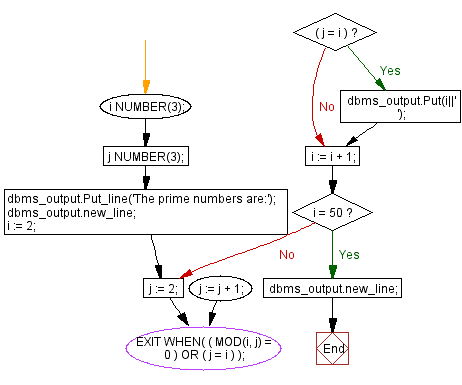﻿ PL/SQL Control Statement: Print the prime numbers between 1 to 50 - w3resource# PL/SQL Control Statement Exercises: Print the prime numbers between 1 to 50

## PL/SQL Control Statement: Exercise-27 with Solution

Write a program in PL/SQL to print the prime numbers between 1 to 50.

Sample Solution:

PL/SQL Code:

``````DECLARE
i NUMBER(3);
j NUMBER(3);
BEGIN
dbms_output.Put_line('The prime numbers are:');
dbms_output.new_line;
i := 2;
LOOP
j := 2;
LOOP
EXIT WHEN( ( MOD(i, j) = 0 )
OR ( j = i ) );
j := j + 1;
END LOOP;
IF( j = i )THEN
dbms_output.Put(i||'   ');
END IF;
i := i + 1;
exit WHEN i = 50;
END LOOP;
dbms_output.new_line;
END;
/
```
```

Flowchart:Sample Output:

```The prime numbers are:
2   3   5   7   11   13   17   19   23   29   31   37   41   43   47

PL/SQL procedure successfully completed.
```

Improve this sample solution and post your code through Disqus

What is the difficulty level of this exercise?

﻿